# RD Sharma Solutions For Class 12 Maths Exercise 19.15 Chapter 19 Indefinite Integrals

RD Sharma Solutions for Class 12 Maths Exercise 19.15 Chapter 19 Indefinite Integrals is provided here. This exercise covers the evaluation of integrals of the type

$$\begin{array}{l}\int \frac{1}{ax^{2} + bx + c} \ dx\end{array}$$
. To evaluate these types of integrals we express
$$\begin{array}{l}{ax^{2} + bx + c}\end{array}$$
as the sum or difference of two squares. The solutions have been created by our expert team at BYJU’S. The main aim to create such a vital resource for students is to help them self analyse their areas of weaknesses. It also paves the way for the students to compare the solutions they gave for the questions in RD Sharma textbook and check how far they could answer.

The PDF of RD Sharma Solutions for Class 12 is the best guide students can rely on and also to build confidence in solving problems. It provides students with a strong conceptual knowledge too. Students can explore the key points in presenting a solution to a problem.

## Download the PDF of RD Sharma Solutions For Class 12 Chapter 19 – Indefinite Integrals Exercise 19.15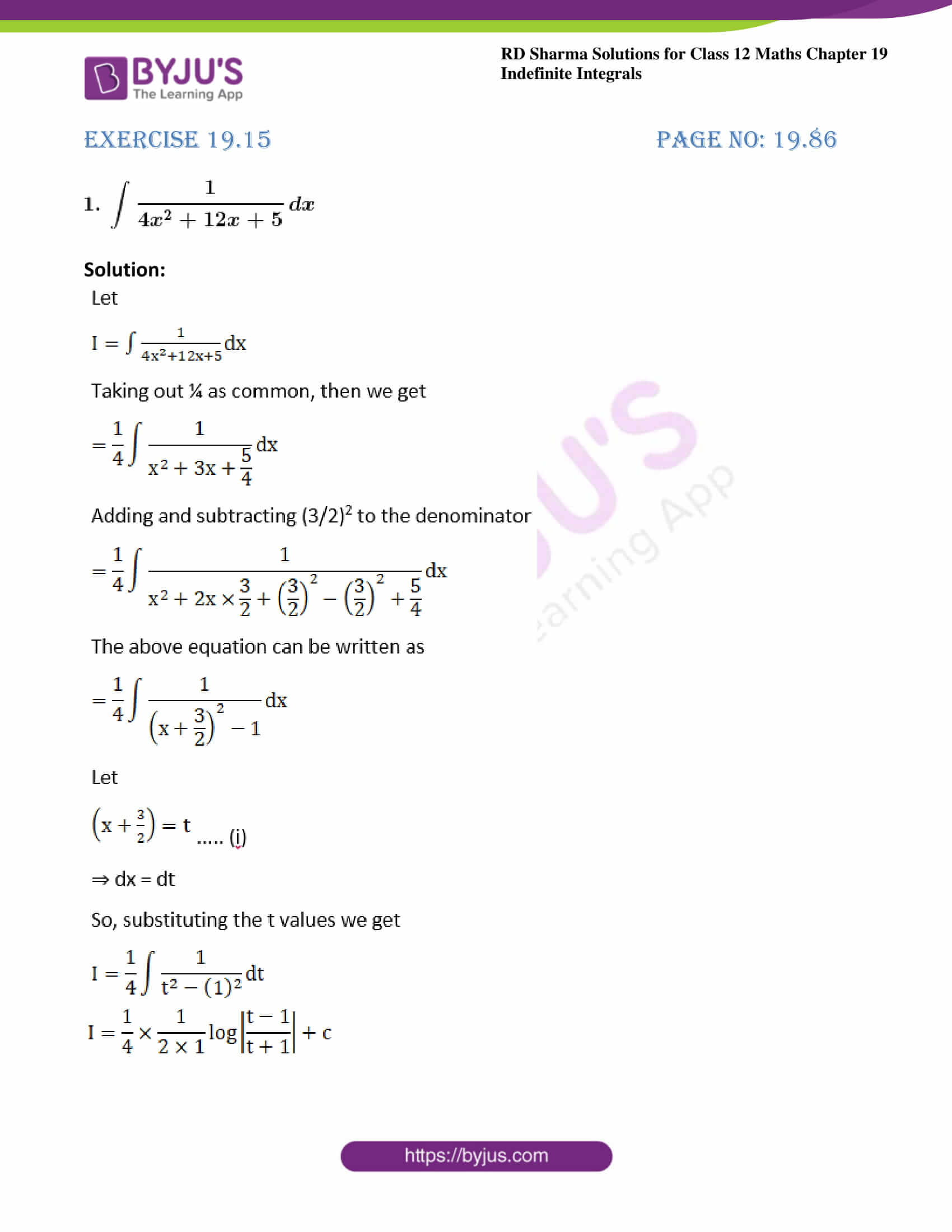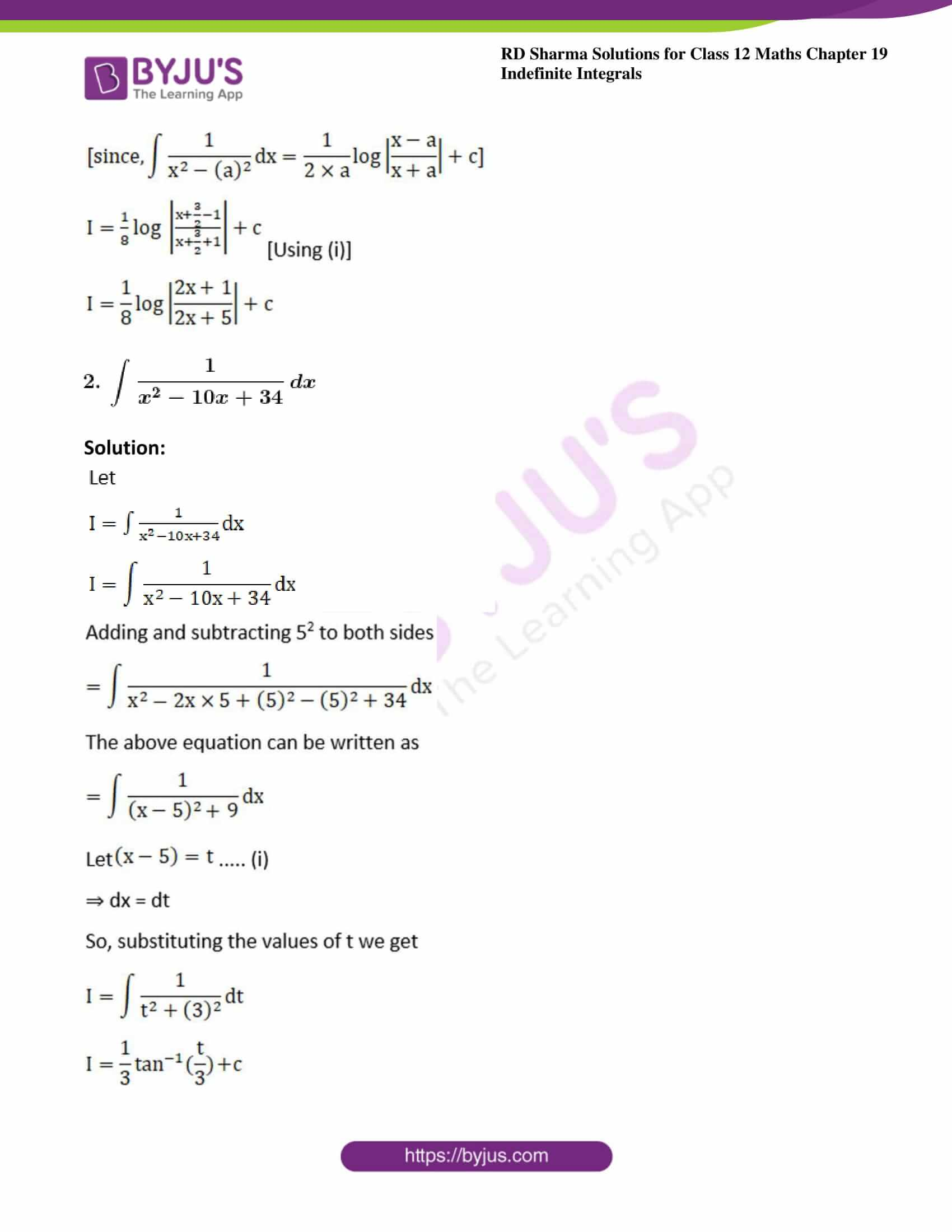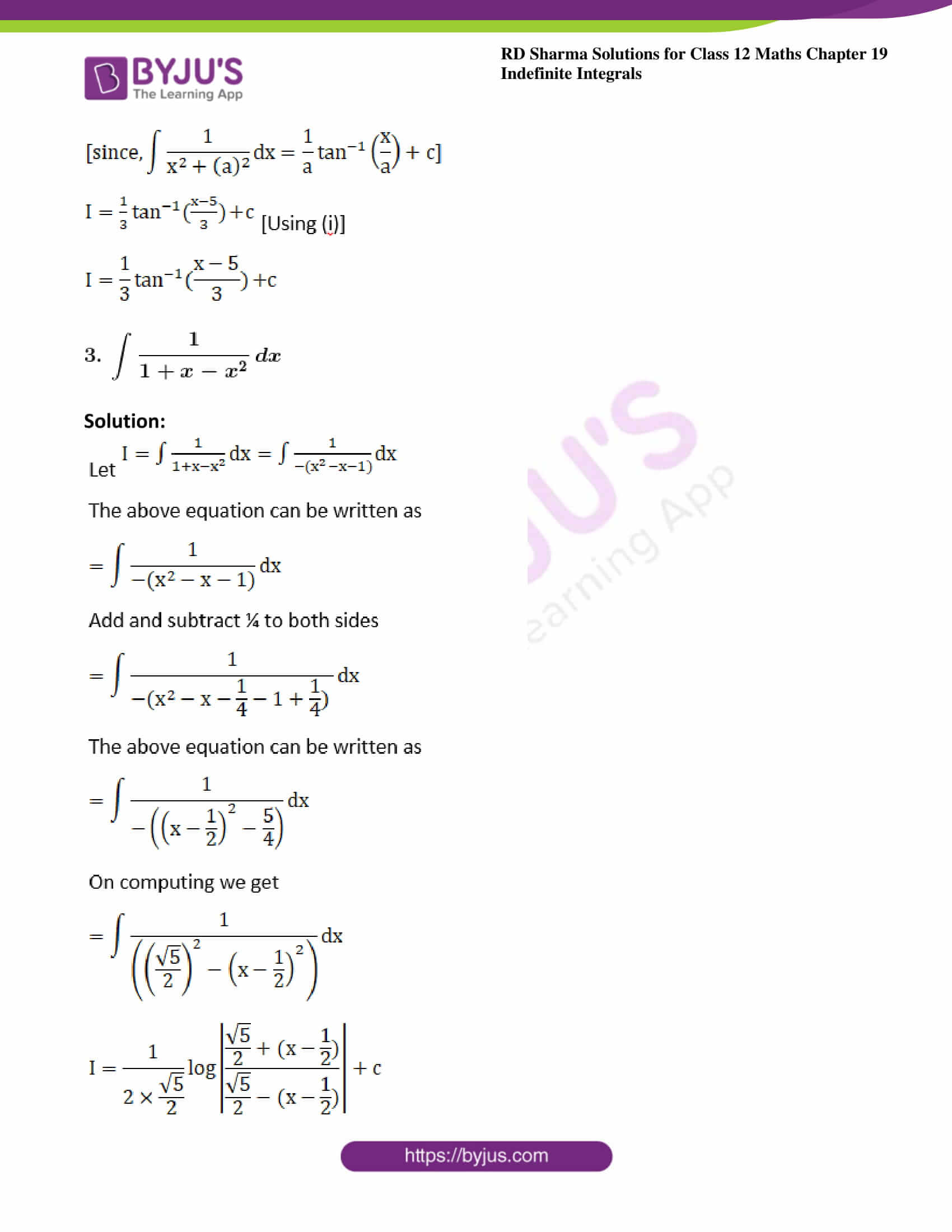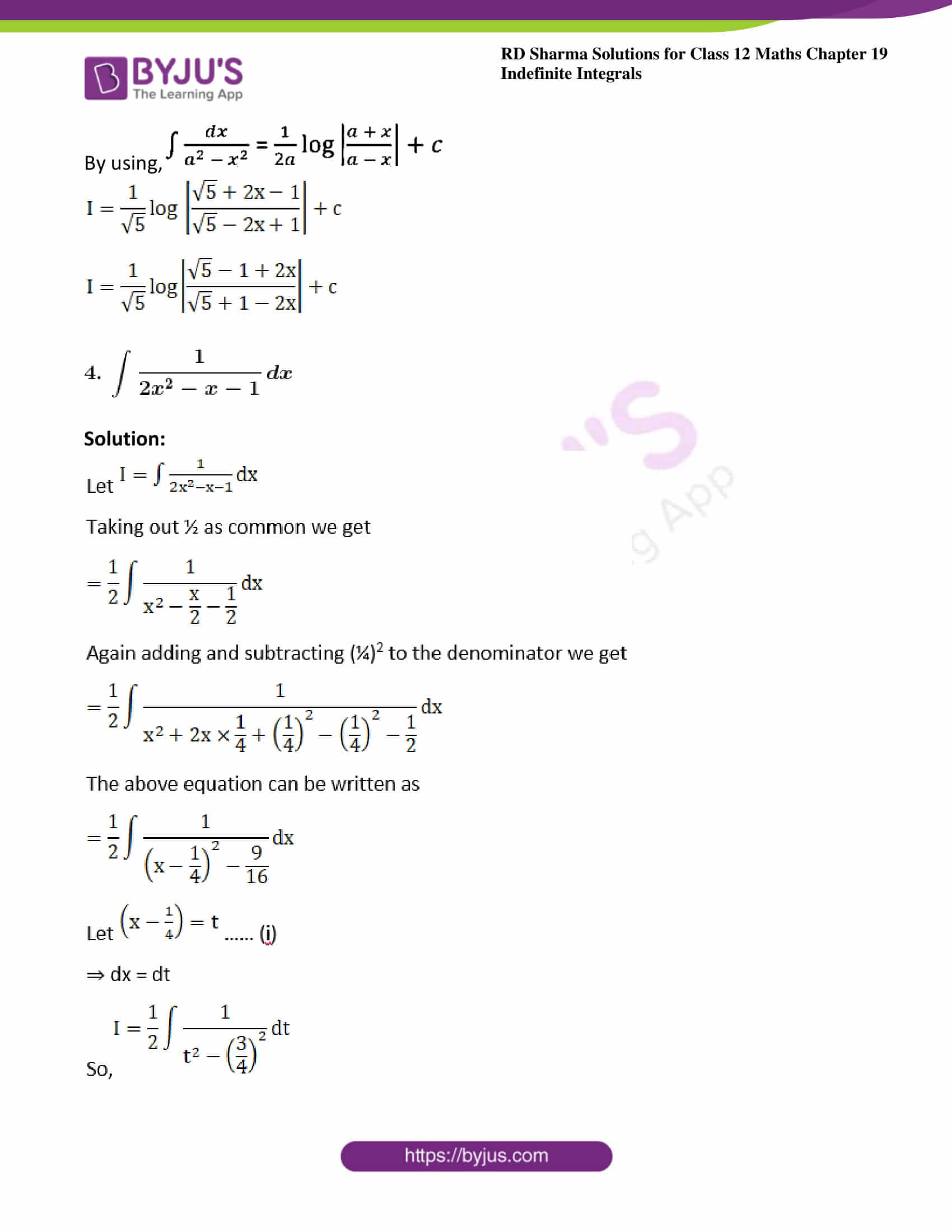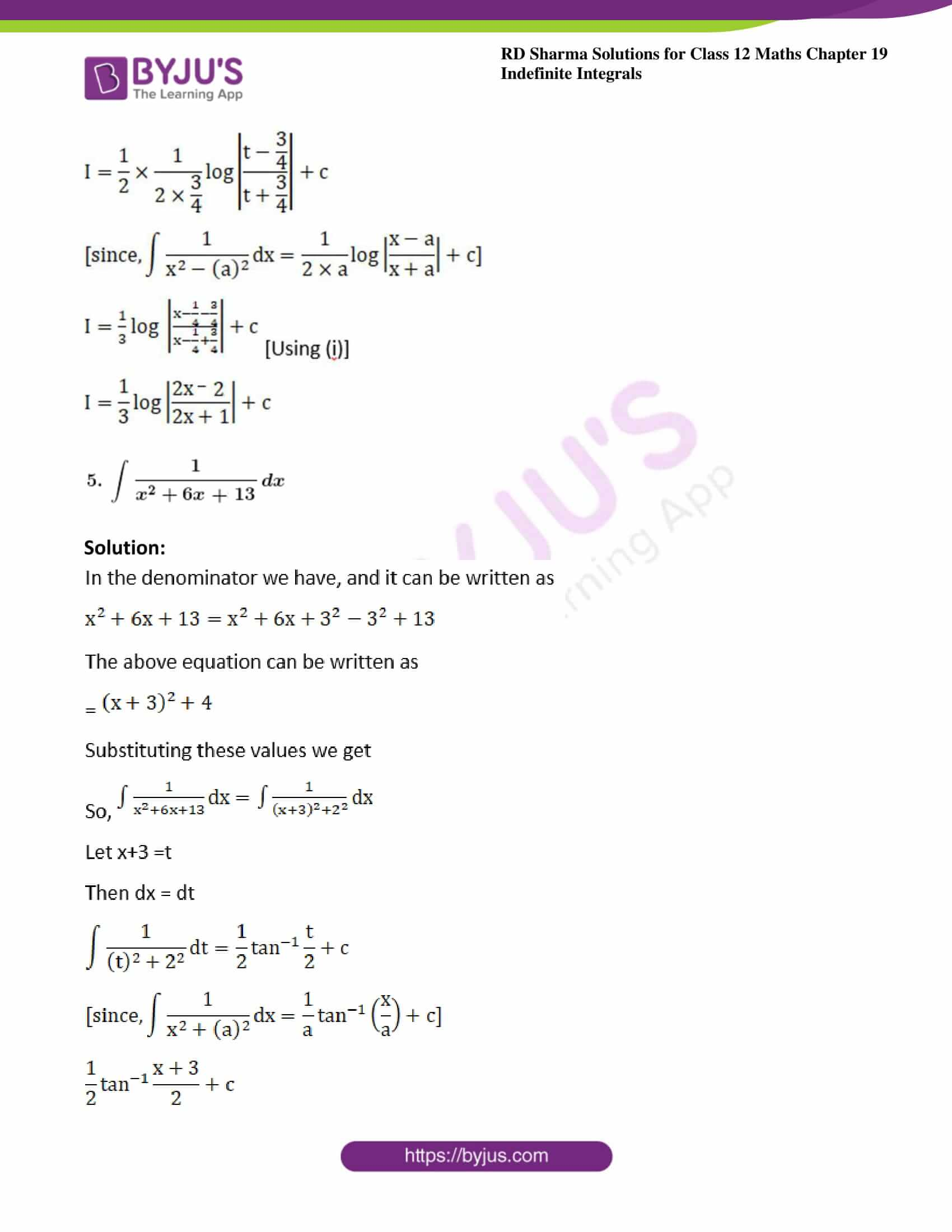### Exercise 19.15 Page No: 19.86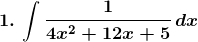Solution: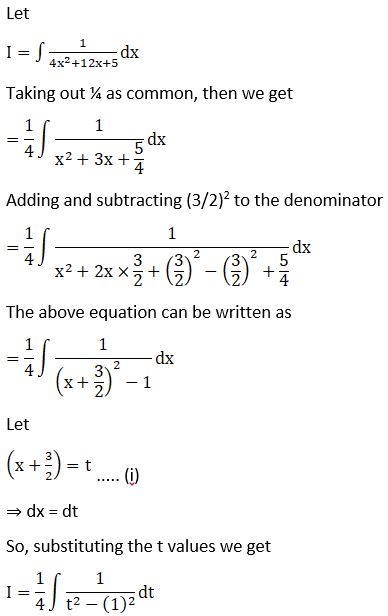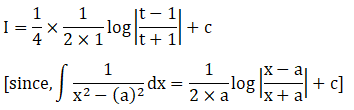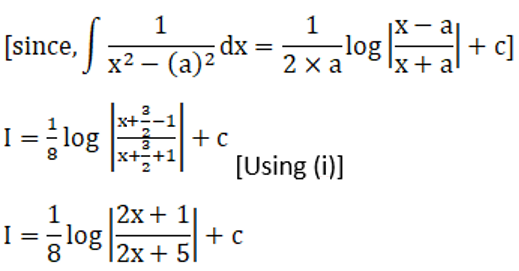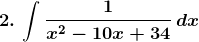Solution: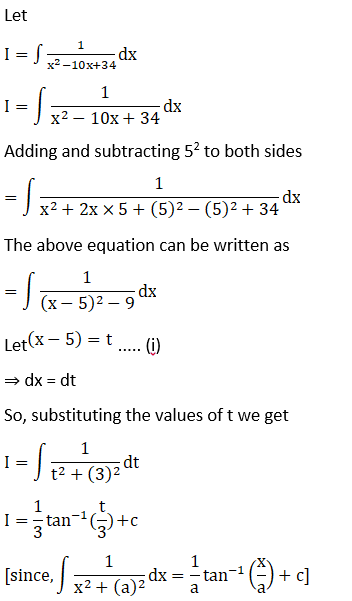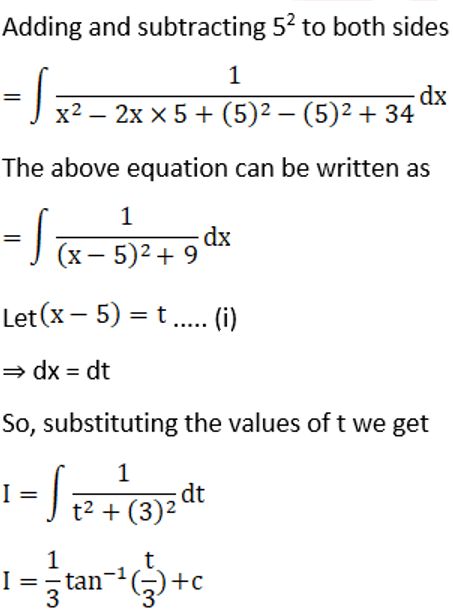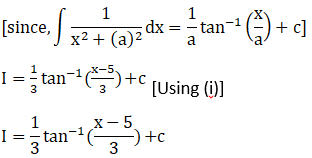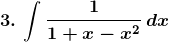Solution: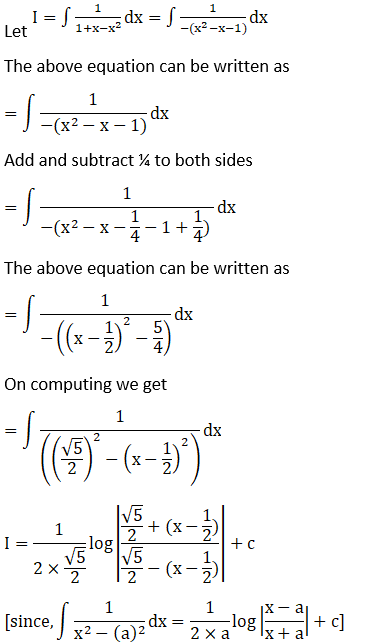By using,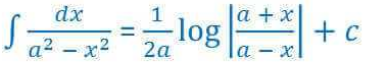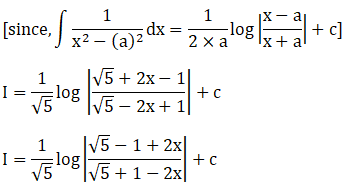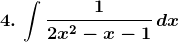Solution: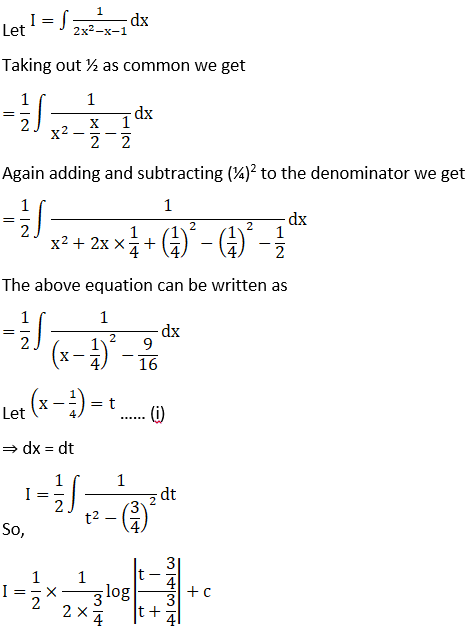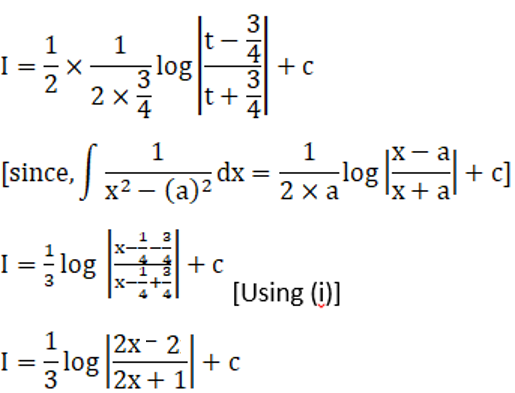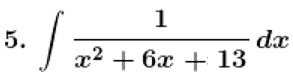Solution: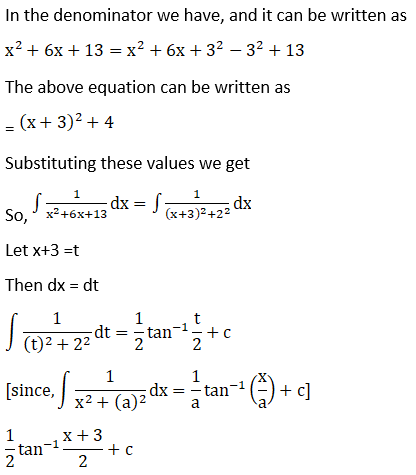### Access other exercises of RD Sharma Solutions For Class 12 Chapter 19 – Indefinite Integrals

Exercise 19.1 Solutions

Exercise 19.2 Solutions

Exercise 19.3 Solutions

Exercise 19.4 Solutions

Exercise 19.5 Solutions

Exercise 19.6 Solutions

Exercise 19.7 Solutions

Exercise 19.8 Solutions

Exercise 19.9 Solutions

Exercise 19.10 Solutions

Exercise 19.11 Solutions

Exercise 19.12 Solutions

Exercise 19.13 Solutions

Exercise 19.14 Solutions

Exercise 19.16 Solutions

Exercise 19.17 Solutions

Exercise 19.18 Solutions

Exercise 19.19 Solutions

Exercise 19.20 Solutions

Exercise 19.21 Solutions

Exercise 19.22 Solutions

Exercise 19.23 Solutions

Exercise 19.24 Solutions

Exercise 19.25 Solutions

Exercise 19.26 Solutions

Exercise 19.27 Solutions

Exercise 19.28 Solutions

Exercise 19.29 Solutions

Exercise 19.30 Solutions

Exercise 19.31 Solutions

Exercise 19.32 Solutions